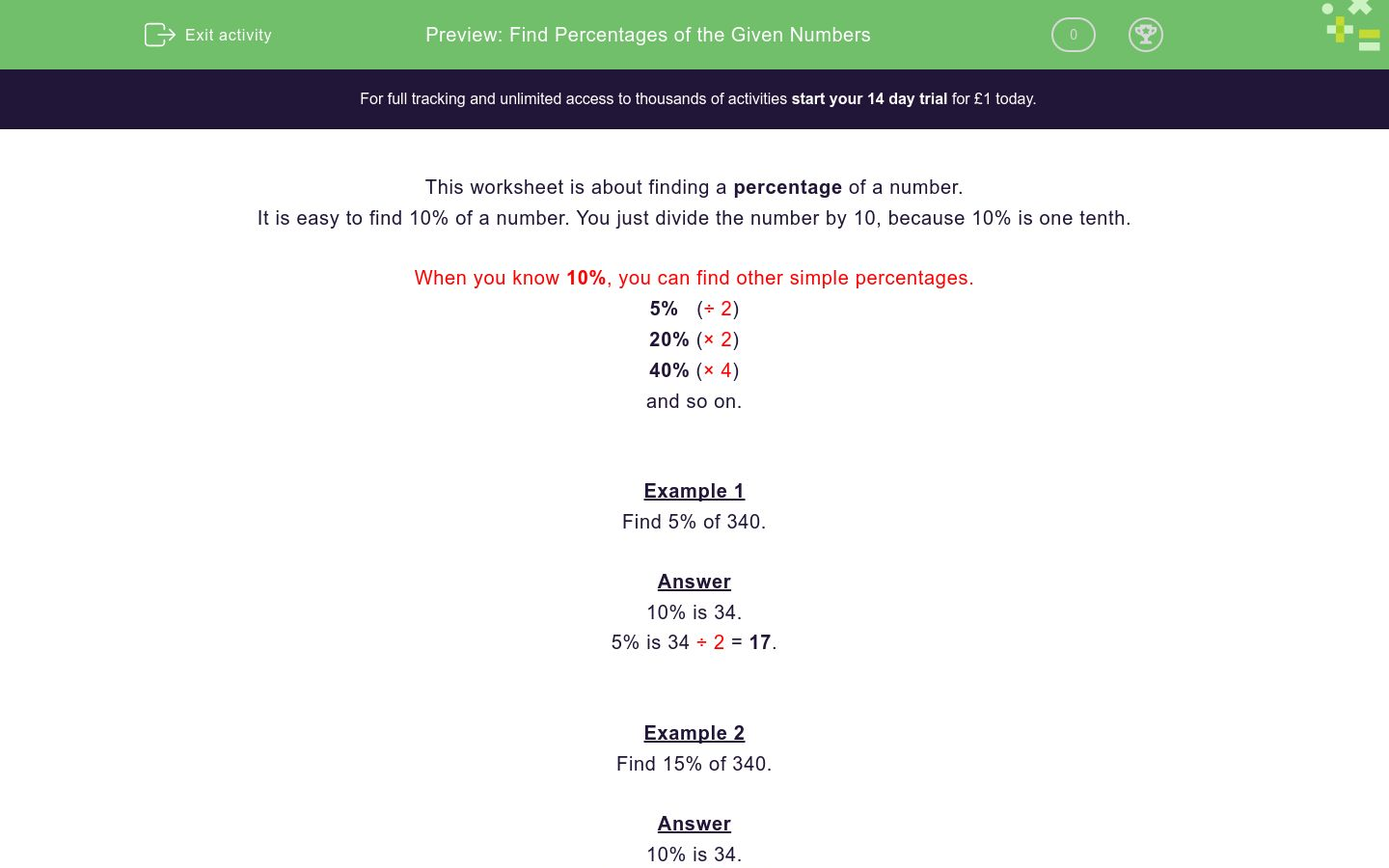# Find Percentages of the Given Numbers

In this worksheet, students find a percentage of a given number.Key stage:  KS 2

Curriculum topic:  Ratio and Proportion

Curriculum subtopic:  Solve Percentage Problems

Difficulty level:### QUESTION 1 of 10

This worksheet is about finding a percentage of a number.

It is easy to find 10% of a number. You just divide the number by 10, because 10% is one tenth.

When you know 10%, you can find other simple percentages.

5%   (÷ 2)

20% (× 2)

40% (× 4)

and so on.

Example 1

Find 5% of 340.

10% is 34.

5% is 34 ÷ 2 = 17.

Example 2

Find 15% of 340.

10% is 34.

5% is 34 ÷ 2 = 17.

Add these to get 15% which will be 34 + 17 = 51.

Find

10% of 70

Find

20% of 80

Find

10% of 320

Find

20% of 320

Find

10% of 520

Find

5% of 520

Find

5% of 180

Find

15% of 80

Find

30% of 360

Find

15% of 360

• Question 1

Find

10% of 70

7
EDDIE SAYS
70 ÷ 10 = 7
• Question 2

Find

20% of 80

16
EDDIE SAYS
10% of 80 is 8.
20% is double this.
• Question 3

Find

10% of 320

32
EDDIE SAYS
320 ÷ 10 = 32
• Question 4

Find

20% of 320

64
EDDIE SAYS
10% is 32.
20% is double this.
• Question 5

Find

10% of 520

52
EDDIE SAYS
520 ÷ 10 = 52
• Question 6

Find

5% of 520

26
EDDIE SAYS
10% is 52.
5% is half of this.
• Question 7

Find

5% of 180

9
EDDIE SAYS
10% is 18.
5% is half this.
• Question 8

Find

15% of 80

12
EDDIE SAYS
10% is 8.
5% is 4.
• Question 9

Find

30% of 360

108
EDDIE SAYS
10% is 36.
30% is three times this.
• Question 10

Find

15% of 360